Olympiad Test Level 1: Logical Reasoning- 2

# Olympiad Test Level 1: Logical Reasoning- 2

Test Description

## 10 Questions MCQ Test GK Olympiad for Class 6 | Olympiad Test Level 1: Logical Reasoning- 2

Olympiad Test Level 1: Logical Reasoning- 2 for Class 6 2022 is part of GK Olympiad for Class 6 preparation. The Olympiad Test Level 1: Logical Reasoning- 2 questions and answers have been prepared according to the Class 6 exam syllabus.The Olympiad Test Level 1: Logical Reasoning- 2 MCQs are made for Class 6 2022 Exam. Find important definitions, questions, notes, meanings, examples, exercises, MCQs and online tests for Olympiad Test Level 1: Logical Reasoning- 2 below.
Solutions of Olympiad Test Level 1: Logical Reasoning- 2 questions in English are available as part of our GK Olympiad for Class 6 for Class 6 & Olympiad Test Level 1: Logical Reasoning- 2 solutions in Hindi for GK Olympiad for Class 6 course. Download more important topics, notes, lectures and mock test series for Class 6 Exam by signing up for free. Attempt Olympiad Test Level 1: Logical Reasoning- 2 | 10 questions in 20 minutes | Mock test for Class 6 preparation | Free important questions MCQ to study GK Olympiad for Class 6 for Class 6 Exam | Download free PDF with solutions
 1 Crore+ students have signed up on EduRev. Have you?
Olympiad Test Level 1: Logical Reasoning- 2 - Question 1

### Which of the following is a prime number?

Detailed Solution for Olympiad Test Level 1: Logical Reasoning- 2 - Question 1

Clearly, 97 is a prime number.

Olympiad Test Level 1: Logical Reasoning- 2 - Question 2

### What least number should be added to 1056, so that the sum is completely divisible by 23?

Detailed Solution for Olympiad Test Level 1: Logical Reasoning- 2 - Question 2

(1056/23) givess remainder 21 21 + 2 = 23, So we need to add 2

Olympiad Test Level 1: Logical Reasoning- 2 - Question 3

### One side of rectangular field is 15 meter and one of its diagonals is 17 meters. Then find the area of the field.

Detailed Solution for Olympiad Test Level 1: Logical Reasoning- 2 - Question 3

We know h2 = b2 + h2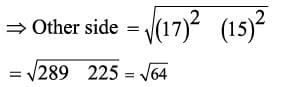= 8 meter
Area = Length x Breadth
= 15 x 8 m2 = 120m2

Olympiad Test Level 1: Logical Reasoning- 2 - Question 4

₹120 are divided among A, B, C such that A’s share is ₹ 20 more than B’s and ₹ 20 less than C’s. What is B’s share?

Detailed Solution for Olympiad Test Level 1: Logical Reasoning- 2 - Question 4

Let C = x. Then A = (x - 20)  and B = (x - 40).
x + x - 20 + x - 40 = 120 Or x = 60.
A : B : C = 40 : 20 : 60 = 2 : 1 : 3.
B’s share = ? 120 x (1/6) = ₹ 20

Olympiad Test Level 1: Logical Reasoning- 2 - Question 5

ABCD is a rhombus with diagonals AC and BD.Then, which one among the following is correct?

Detailed Solution for Olympiad Test Level 1: Logical Reasoning- 2 - Question 5

AC and BD bisect each other and perpendicular to each other. ABCD is a rhombus.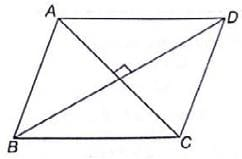Therefore, AB = BC = CD = DA
And diagonals bisect each other at right angles.

Olympiad Test Level 1: Logical Reasoning- 2 - Question 6

Three numbers are in the ratio 1:2:3. It the sum of their cubes is 98784, then the numbers are

Detailed Solution for Olympiad Test Level 1: Logical Reasoning- 2 - Question 6

Let the numbers be x, 2x, 3x.
Then (x)3 +(2x)3 + (3x)3 = 98784
x3 + 8x3 + 27x3 = 98784
36x3 = 98784
x3 = 2744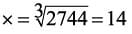Hence the numbers are 14,28,42

Olympiad Test Level 1: Logical Reasoning- 2 - Question 7

A piece of paper is folded and cut. from the figures given, indicate how it will appear when opened
Question Figure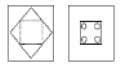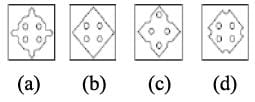Detailed Solution for Olympiad Test Level 1: Logical Reasoning- 2 - Question 7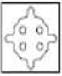Olympiad Test Level 1: Logical Reasoning- 2 - Question 8

Identify the figure that completes the pattern.
Question Figure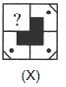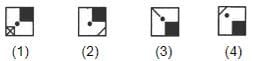Detailed Solution for Olympiad Test Level 1: Logical Reasoning- 2 - Question 8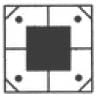Olympiad Test Level 1: Logical Reasoning- 2 - Question 9

Directions: Select the related word/letter/ number from the given alternatives.

ABDE : PQST :: MNPQ : ?

Detailed Solution for Olympiad Test Level 1: Logical Reasoning- 2 - Question 9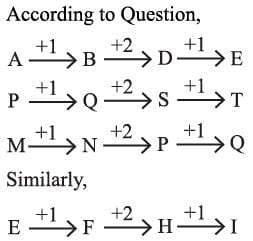Olympiad Test Level 1: Logical Reasoning- 2 - Question 10

Directions: Select the related word/letter/ number from the given alternatives.

64 : 100 : : 16 : ?

Detailed Solution for Olympiad Test Level 1: Logical Reasoning- 2 - Question 10

The pattern is:
64 = (8)2
100 = (8 + 2)2 = (10)2
16 = (4)2
36 = (4 + 2)2 = (6)2

## GK Olympiad for Class 6

22 docs|58 tests
 Use Code STAYHOME200 and get INR 200 additional OFF Use Coupon Code
Information about Olympiad Test Level 1: Logical Reasoning- 2 Page
In this test you can find the Exam questions for Olympiad Test Level 1: Logical Reasoning- 2 solved & explained in the simplest way possible. Besides giving Questions and answers for Olympiad Test Level 1: Logical Reasoning- 2, EduRev gives you an ample number of Online tests for practice

22 docs|58 tests